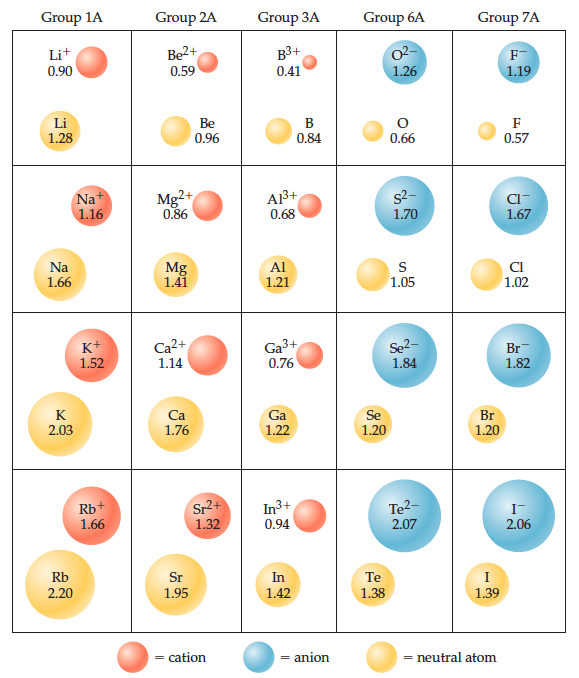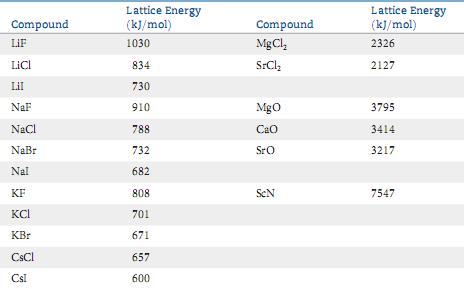# Problem: Consider the ionic compounds KF, NaCl, NaBr, and LiCl. Use ionic radii (from the figure below) to estimate the cation–anion distance for each compound. Based on your answers, arrange these four compounds in order of decreasing lattice energy.Check your predictions with the experimental values of lattice energy from the table. Are the predictions from ionic radii correct?

###### FREE Expert Solution
91% (458 ratings)
###### Problem Details

Consider the ionic compounds KF, NaCl, NaBr, and LiCl. Use ionic radii (from the figure below) to estimate the cation–anion distance for each compound. Based on your answers, arrange these four compounds in order of decreasing lattice energy.Check your predictions with the experimental values of lattice energy from the table. Are the predictions from ionic radii correct?